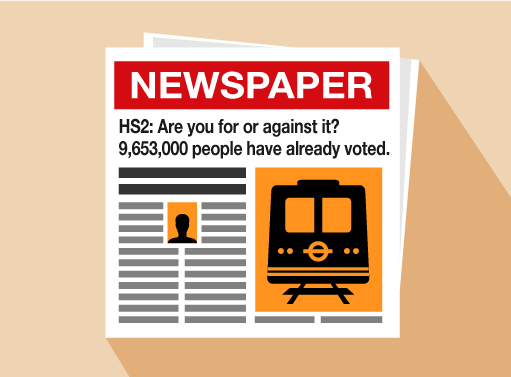Everyday maths for Construction and Engineering 1

Start this free course now. Just create an account and sign in. Enrol and complete the course for a free statement of participation or digital badge if available.

Free course

# 1.3 Working with whole numbers

The following activities cover everything in the whole numbers section. As you attempt the activities, look for key words to identify what the question is asking you to do.

## Activity 3: Looking at numbers

1. Look at this newspaper headline:• a.Write down the number in millions.
• b.Write down the number in thousands.
• c.Look at the details below. Which side has received the most votes for the HS2 project?
• For the project: 4,850,000 votes
• Against the project: 4,803,000 votes
1. Look at the data in the following table. It gives the temperatures of five cities on a Monday in January.
CityTemperature
London0°C
Paris–1°C
Delhi28°C
Moscow–10°C
•

• a.Which city was the coldest?
• b.Which city was the warmest?
• c.How many cities have a temperature below 5°C?
1. You buy a pair of work boots for £24 and a dust jacket for £18. How much do you spend altogether?
1. You have £48. You spend £26. How much do you have left?

1. The answers are as follows:
• a.9 million
• b.653 thousand
• c.For the project
2. The answers are as follows:
• a.Moscow
• b.Delhi
• c.London, Paris and Moscow
3. £24 + £18 = £42
4. £48 – £26 = £22

## Activity 4: Using multiplication and division

You can use a calculator in this activity.

1. What are the answers to these sums?
• a.6 × 4
• b.3 × 9
• c.5 × 7
• d.36 ÷ 9
• e.48 ÷ 6
• f.15 ÷ 3
2. Hinges come in boxes of 10. There are 25 boxes in a crate. How many hinges are there in one crate?
3. A modified-car show is selling tickets at £19 for adults and £11 for children. How much would it cost for two adults and two children to go?

1. The answers are as follows:
• a.6 ×4 = 24
• b.3 × 9 = 27
• c.5 × 7 = 35
• d.36 ÷ 9 = 4
• e.48 ÷ 6 = 8
• f.15 ÷ 3 = 5
2. 10 × 25 = 250 hinges.
3. 2 × 19 = £38

11 × 2 = £22

22 + 38 = £60

It would cost £60 to go to the modified car show.

FSM_SSC_1